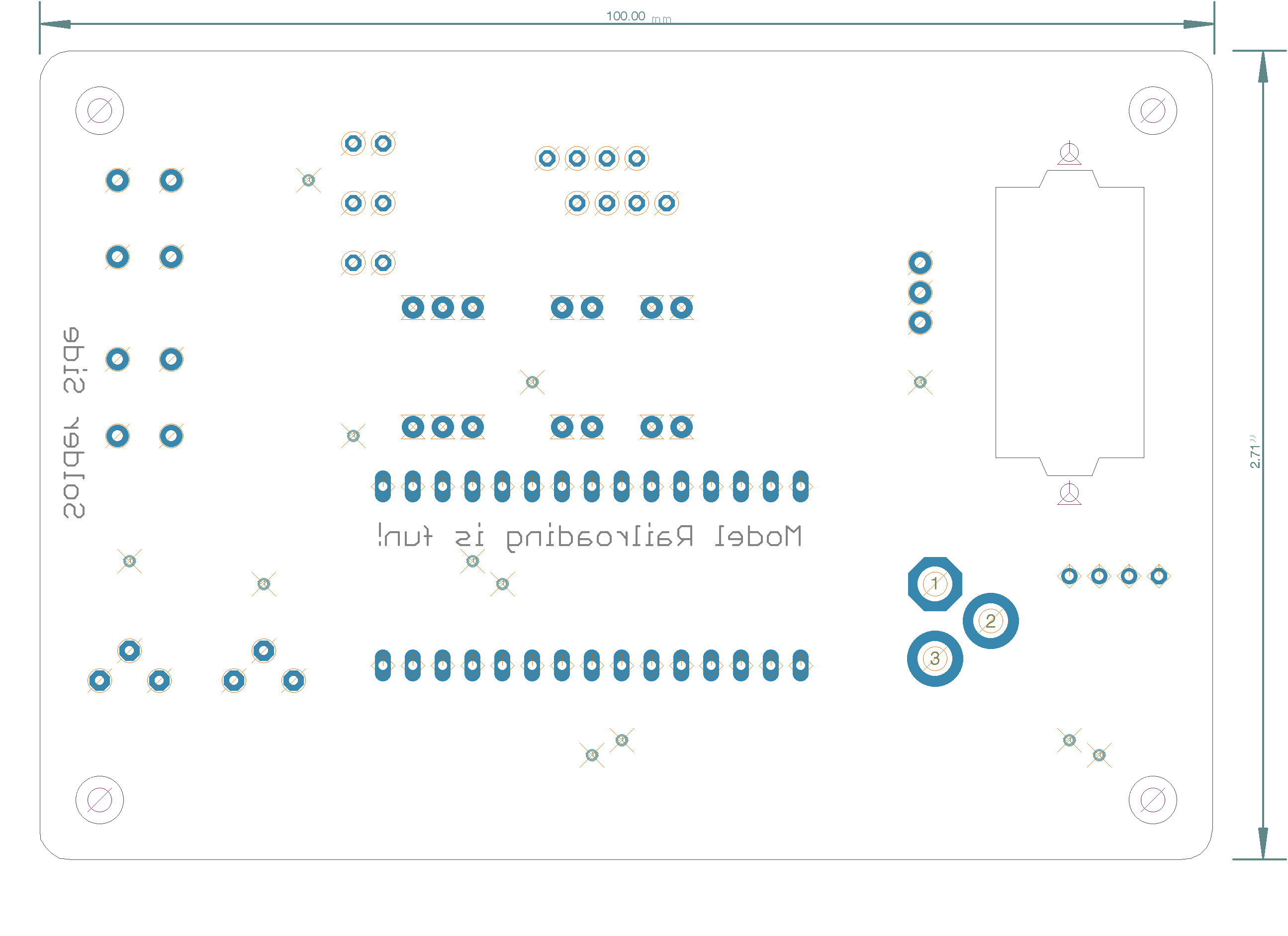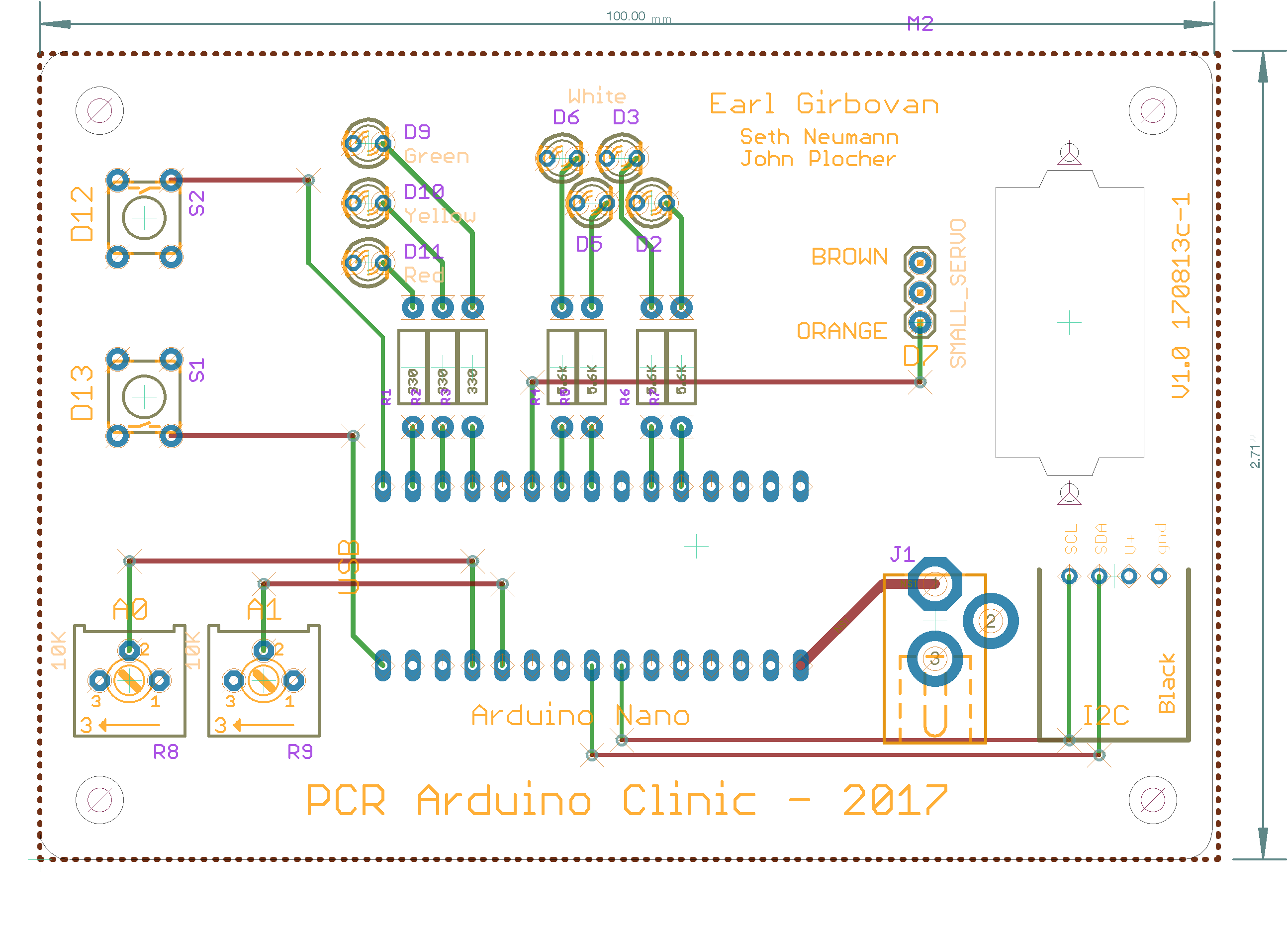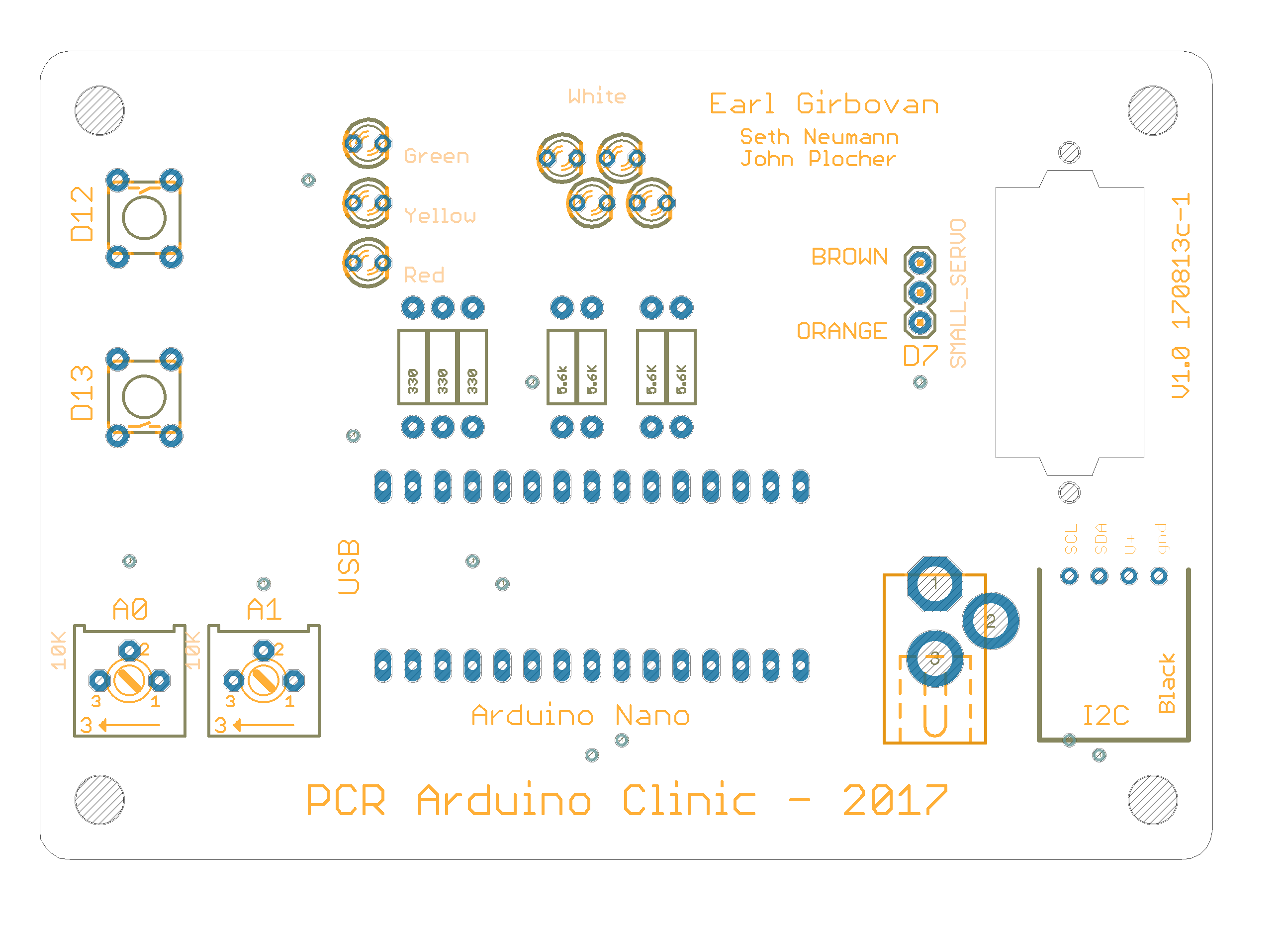MRCS-PCR-Clinic

## MRCS-PCR-Clinic Version 1.0

First built: 2017-08

170812c-Mod1

• Enlarged servo mounting cutout to fit servo with adhesive labelBot SilkBoardTop Silk

## MRCS-PCR-Clinic.bom

Parts Value Package Quantity Library Type/Feeder
R4, R5, R6, R7 5.6K AXIAL-0.4 4x SparkFun-Resistors PTH
R8, R9 10K 3386P 2x bourns_test PTH
R1, R2, R3 330 AXIAL-0.4 3x SparkFun-Resistors PTH
U\$10   BOARD-DINRAIL-2.7IN 1x SPCoast-minimal PTH
J1   DCJ0202 1x SPCoast PTH
U\$11   SERVO-9G 1x SPCoast PTH
D9 Green LED3MM 1x SPCoast PTH
U\$9 I2CRA I2C 1x SPCoast-minimal PTH
U\$1 NANO NANO 1x Arduino-clone-scn PTH
D11 Red LED3MM 1x SPCoast PTH
M2 SMALL_SERVO 1X03 1x SparkFun-Electromechanical PTH
S1, S2 SWITCH-MOMENTARY-2PTH TACTILE-PTH 2x SparkFun-Electromechanical PTH
D2, D3, D5, D6 White LED3MM 4x SPCoast PTH
D10 Yellow LED3MM 1x SPCoast PTH

## Documentation

Project board for PCR-NMRA Arduino Programming class by Earl Girbovan and Seth Neumann

The Project Board includes examples of inputs (push buttons, potentiometers) and outputs (LEDs, an SG90 Servo) that are often found in model railroad projects. Since the clinic is about learning the Arduino Integrated Development Environment (IDE) and learning the Arduino “Processing” language, the board is offered assembled so you can start right away without the need to do assembly. Connectors are provided for the Nano (included with the A&T version), external power and ground, and the i/o pins not used on the boards. Pads are provided for an I2C connection if you want to expand your project using our IOX16 and IOX32.

``````/* Blink

This program blinks an LED on and off

*/

const int LED2 = 2;  //D2

void setup()
{
pinMode( LED2, OUTPUT );

}

void loop()
{
digitalWrite( LED2, HIGH );
delay( 1000);
digitalWrite( LED2, LOW );
delay ( 1000 );

}

``````

## Fire_simulator

``````/* LED Fire Effect
Uses two amber and one red LED to simulate fire
From the instructables.com web site, slightly modified
*/

int ledPin1 =  9;
int ledPin2 = 10;
int ledPin3 = 11;

void setup()
{
pinMode(ledPin1, OUTPUT);
pinMode(ledPin2, OUTPUT);
pinMode(ledPin3, OUTPUT);
}

void loop()
{
analogWrite(ledPin1, random(135, 255));
analogWrite(ledPin2, random(135, 255));
analogWrite(ledPin3, random(135, 255));
delay(random(1, 100));
}

``````

``````/*

Ties together the blinking lights and servomotor sweep,
The servo simulates a crossing arm.

The sequence is a bit stylized to keep the code manageable
for the clinic.
*/

#include <Servo.h>
Servo Servo1;

int pos;
int MaxPos   = 90;   // max position in degrees
int ServoPos;        // where the servo is in it's sweep
int StepTime = 90;   // time between steps
int ServoTime;       // time since last step

const int TriggerPin = 12;  //Digitial input D12
int Trigger;

int LEDTime;
int Delta = 90;    // number of msec for each loop iteration
int LEDPin = 2;
boolean LEDState;

void setup()
{
pinMode( TriggerPin, INPUT_PULLUP);
//tell the Arduino & the servo driver that the servo is connected to pin 7
Servo1.attach(7);
Servo1.write( 0 );   // ensure the crossing gate is raised
ServoPos = 0;
LEDState = false;    // LED starts off
pinMode ( LEDPin, OUTPUT );
digitalWrite ( LEDPin, HIGH );
} // end of setup

void loop()
{
//poll, looking for a LOW to start the sequence
do
{
}
while( Trigger == HIGH );

//start the sequence
ServoTime  = StepTime;   // initialize the crossing arm timer
LEDTime    = 0;          // go into the routine with the LED off, so it will turn on
LEDState   = false;
ServoPos   = 0;         // crossing arm raised position
do
{
// see if we need to change the stat of the LED
LEDTime = LEDTime - Delta;
if( LEDTime <= 0 )
{
LEDState = !LEDState;  //LED to other state; on or off
if (LEDState)
{
digitalWrite ( LEDPin, LOW );
}
else
{
digitalWrite ( LEDPin, HIGH );
}
}

//see if we need to change the state of the crossing arm
ServoTime = ServoTime - Delta;
if( ServoTime <= 0 )
{
//if arm not at down position, move it
if( ServoPos < MaxPos )
{
ServoPos++;
Servo1.write( ServoPos );
// and reset the timer for the next step
ServoTime = StepTime;
}
}
delay ( Delta );
}
while ( Trigger == LOW );

//switch has been released, turn off the light and raise the arm
digitalWrite( LEDPin, HIGH );

for( int pos = ServoPos; pos >=1; pos--)
{
Servo1.write(pos);
delay( StepTime );
}

} // end of loop

``````

``````/*

Ties together the blinking lights and servomotor sweep,
The servo simulates a crossing arm.

The sequence is a bit stylized to keep the code manageable
for the clinic.
*/

#include <Servo.h>
Servo Servo1;

int pos;
int MaxPos   = 90;   // max position in degrees
int ServoPos;        // where the servo is in it's sweep
int StepTime = 90;   // time between steps
int ServoTime;       // time since last step

const int TriggerPin = 12;  //Digitial input D12
int Trigger;

int LEDTime;
int Delta = 90;    // number of msec for each loop iteration
int LEDPin = 2;
boolean LEDState;

void setup()
{
pinMode( Triggerpin, INPUT_PULLUP);
//tell the Arduino & the servo driver that the servo is connected to pin 7
Servo1.attach(7);
Servo1.write( 0 );   // ensure the crossing gate is raised
ServoPos = 0;
LEDState = false;    // LED starts off
pinMode ( LEDPin, OUTPUT );
digitalWrite ( LEDPin, HIGH );
} // end of setup

void loop()
{
//poll, looking for a LOW to start the sequence
do
{
}
while( Trigger == HIGH );

//start the sequence
ServoTime  = StepTime;   // initialize the crossing arm timer
LEDTime    = 0;          // go into the routine with the LED off, so it will turn on
LEDState   = false;
ServoPos   = 0;         // crossing arm raised position
do
{
// see if we need to change the stat of the LED
LEDTime = LEDTime - Delta;
if( LEDTime <= 0 )
{
LEDState = !LEDState;  //LED to other state; on or off
if (LEDState)
{
digitalWrite ( LEDPin, LOW );
}
else
{
digitalWrite ( LEDPin, HIGH );

}

//see if we need to change the state of the crossing arm
ServoTime = ServoTime - Delta;
if( ServoTime <= 0 )
{
//if arm not at down position, move it
if( ServoPos < MaxPos )
{
ServoPos++;
Servo1.write( ServoPos );
// and reset the timer for the next step
ServoTime = StepTime;
}
}
delay ( Delta );
}
while ( Trigger = LOW );

//switch has been released, turn off the light and raise the arm
digitalWrite( LEDPin, HIGH );

for( int pos = ServoPos; pos >=1; pos--)
{
Servo1.write(pos);
delay( StepTime );
}

} // end of loop

``````

## Intermittent_Fire_simulator

``````/* LED Fire Effect
Uses two amber and one red LED to simulate fire
From the instructables.com web site

Included a WHILE loop, so that the effect turns on and off.
*/

int ledPin1 =  9;
int ledPin2 = 10;
int ledPin3 = 11;
int OnTime;
int OffTime;
int i;

void setup()
{
pinMode(ledPin1, OUTPUT);
pinMode(ledPin2, OUTPUT);
pinMode(ledPin3, OUTPUT);
}

void loop()
{
OnTime = random( 1000, 5000 );
while( OnTime > 0 )
{
analogWrite(ledPin1, random(120)+135);
analogWrite(ledPin2, random(120)+135);
analogWrite(ledPin3, random(120)+135);
i = random(1, 100);
delay( i );
OnTime = OnTime - i;
}

// off time, ensure the LEDs are turned off
analogWrite(ledPin1, 255 );
analogWrite(ledPin2, 255 );
analogWrite(ledPin3, 255 );
OffTime = random ( 1000, 3000 );
delay ( OffTime );
}

``````

## PCR_test_and_demo

``````/*

servo Sweep is by BARRAGAN <http://barraganstudio.com>
This example code is in the public domain.

modified 8 Nov 2013
by Scott Fitzgerald
http://arduino.cc/en/Tutorial/Sweep

*/

#include <Servo.h>

// LED setup
#define LED2 2     // LED no PWM
#define LED3 3     // LEDs PWM
#define LED5 5     // LEDs PWM
#define LED6 6     // LEDs PWM
#define LED9  9
#define LED10 10
#define LED11 11
// push button for LED 9
#define BUTTON 12

#define SERVO_PIN  7

#define MAX 255 // PWM maximum
#define MID  96 // PWM mid point, not really but more visible
#define MIN   0 // PWM minimum
#define TICK 10 // clock tick

Servo myservo;  // create servo object to control a servo
// twelve servo objects can be created on most boards

int pos = 0;    // variable to store the servo position

int buttonval = 0; // for push button test -- lites LED 9
int pot0val = 0; // for pot 0, lites LED 10
int pot1val = 0; // for pot 1, lites LED 11

void setup() {
myservo.attach(SERVO_PIN);  // attaches the servo on pin 7 to the servo object

pinMode(LED9, OUTPUT);
pinMode(LED10, OUTPUT);
pinMode(LED11, OUTPUT);
pinMode(BUTTON, INPUT_PULLUP);
}

void loop() {
for (byte i = 0; i < 255; i++){
analogWrite(LED2, i);
analogWrite(LED3, i);
analogWrite(LED5, i);
analogWrite(LED6, i);

delay (TICK);
}
delay (1000);

// analogWrite(LED2, MAX);   // turns the LED on
// analogWrite(LED3, MAX);   // turns the LED on
// analogWrite(LED5, MAX);   // turns the LED on
// analogWrite(LED6, MAX);   // turns the LED on

// delay(TICK);             // waits for a second
// analogWrite(LED2, MID);   // turns the LED on halfway
// analogWrite(LED3, MID);   // turns the LED on halfway
// analogWrite(LED5, MID);   // turns the LED on halfway
// analogWrite(LED6, MID);   // turns the LED on halfway

// delay(TICK);             // waits for a second

// analogWrite(LED2, MIN);   // turns the LED off
// analogWrite(LED3, MIN);   // turns the LED off
// analogWrite(LED5, MIN);   // turns the LED off
// analogWrite(LED6, MIN);   // turns the LED off

//delay(TICK);             // waits for a second

// push button

if (buttonval == LOW){
digitalWrite (LED9,LOW);
} else {
digitalWrite (LED9,HIGH);
}

pot0val = analogRead (0); // first pot
analogWrite (LED10, pot0val/4); // scale the 1024 bit input to 256 bit output
pot1val = analogRead (1); // first pot
analogWrite (LED11, pot1val/4); // scale the 1024 bit input to 256 bit output

delay (10);

// from Banzi example 6B
for(pos = 0; pos <= 180; pos += 1) // goes from 0 degrees to 180 degrees
{                                  // in steps of 1 degree
myservo.write(pos);              // tell servo to go to position in variable 'pos'
delay(15);                       // waits 15ms for the servo to reach the position
}

for(pos = 180; pos>=0; pos-=1)     // goes from 180 degrees to 0 degrees
{
myservo.write(pos);              // tell servo to go to position in variable 'pos'
delay(15);                       // waits 15ms for the servo to reach the position
}
}

``````

## Sweep_servo

``````/*
Servo Sweep

This program slowly sweeps a servo back and forth from 0 to a presettable maximum number of degrees.
*/

#include <Servo.h>

Servo Servo1;

int pos;
int MaxPos = 90;
int DelayTime = 90;

void setup()
{
//tell the Arduino & the servo driver that the servo is connected to pin 7
Servo1.attach(7);
}

void loop()
{
//sweep the servo back and forth
for( pos = 1; pos <=MaxPos; pos++ ) //sweep from 0 to MaxPos in 1 degree steps
{
Servo1.write(pos);
delay(DelayTime);
}
delay( 1000 );

//now sweep it back
for( pos = MaxPos; pos >=1; pos--)  //sweep from MaxPos to 0 in 1 degree steps
{
Servo1.write(pos);
delay(DelayTime);
}
delay( 1000 );
}

``````

## doc

Project board for PCR-NMRA Arduino Programming class by Earl Girbovan and Seth Neumann

The Project Board includes examples of inputs (push buttons, potentiometers) and outputs (LEDs, an SG90 Servo) that are often found in model railroad projects. Since the clinic is about learning the Arduino Integrated Development Environment (IDE) and learning the Arduino “Processing” language, the board is offered assembled so you can start right away without the need to do assembly. Connectors are provided for the Nano (included with the A&T version), external power and ground, and the i/o pins not used on the boards. Pads are provided for an I2C connection if you want to expand your project using our IOX16 and IOX32.

## servo_with_travel_set_by_pots

``````
#include <Servo.h> // servo Sweep is by BARRAGAN <http://barraganstudio.com> This example code is in the public domain.
Servo myservo;     // create servo object to control a servo
// twelve servo objects can be created on most boards

int pos;

//const int LED9 = 9; PWM functionality diabled by using servo library
//const int LED10 = 10; PWM functionality diabled by using servo library

int pot0val = 0; // value for pot read
int pot1val = 0; // value for pot read
int lowpos = 1;  // set it in the range of servo
int highpos = 127;// set it in the range of servo

void setup() {

myservo.attach(7);  // attaches the servo on pin 7 to the servo object

//byte i;
}

void loop() {

pot0val = analogRead (0); // first pot
lowpos = pot0val/6; //scale to 1-~180, this is actually 170 degrees
pot1val = analogRead (1); // second pot
highpos = pot1val/6; //scale to 1-~180
{for ( pos=lowpos; pos<=highpos; pos++){

myservo.write(pos);              // tell servo to go to POSition
delay(20);                       // waits 15ms for the servo to reach the position
}
delay(500); // time to recyle
}

// from Banzi example 6B
}

``````